Multiplying & Dividing

Multiplying and Dividing

 Site: TBAISD Moodle Course: Michigan Algebra I Preview 2012 Book: Multiplying & Dividing Printed by: Guest user Date: Friday, July 30, 2021, 07:08 PM

Introduction

Multiplying and dividing functions will require more steps than adding and subtracting functions. While this topic will be covered more thoroughly in the Polynomial Unit, multiplying of linear terms and dividing by a monomial will be discussed here.

The function notation for multiplying and dividing is very similar to adding and subtracting. When multiplying functions,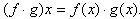When dividing two functions,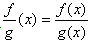, where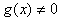because division by zero is an undefined operation in mathematics.

Listen

Multiplying

When multiplying a monomial (or one term expression) by a binomial (or two-term expression), the Distributive Property will be used to multiply the two functions.

Example Given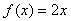and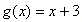, find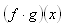.

Step 1. Replace f(x) and g(x) with their equivalent expressions.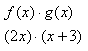Step 2. Use the Distributive Property to multiply and simplify.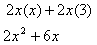For a review of the Distributive Property, select the following link:

Listen

FOIL Method

One method that can be used when multiplying two binomials is the FOIL method. FOIL stands for First, Outer, Inner, and Last. These letters help to remember to multiply each term in the first binomial by each term in the second binomial using the Distributive Property.

Example Given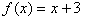and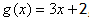, find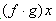.

Step 1. Replace f(x) and g(x) with their equivalent expressions.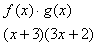Step 2. Multiply the First, Outer, Inner, and Last terms of each binomial.

First + Outer + Inner + Last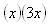+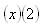+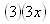+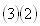Step 3. Simplify the expressions.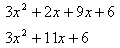For a review of the FOIL method of multiplying, please select the following link:

Listen

Interactive Activity

To solidify your understanding of multiplying two binomials, select the following link:

Practice

Multiplying Binomials Worksheet

Dividing

When dividing two functions,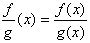, remember that g(x)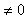since division by zero is undefined. To divide a polynomial by a monomial, divide each term of the polynomial by the monomial. Remember to watch the signs of the terms when dividing and that a number divided by itself is one, do not cancel these terms.

Listen

Example 1

Given f(x) = 5x + 35 and g(x) = 5, find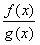.

Step 1. Replace f(x) and g(x) with their equivalent expressions.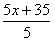Step 2. Divide each term of the polynomial by the monomial.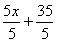Step 3. Simplify.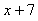Example 2

Given f(x) = 2x2 + 4x + 10 and g(x) = 2, find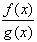.

Step 1. Replace f(x) and g(x) with their equivalent expressions.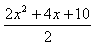Step 2. Divide each term of the polynomial by the monomial.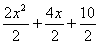Step 3. Simplify.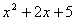Listen

Video Lesson

Guided Practice

To solidify your understanding of function operations, visit the following link to Holt, Rinehart and Winston Homework Help Online. It provides examples, video tutorials and interactive practice with answers available. The Practice and Problem Solving section has two parts. The first part offers practice with a complete video explanation for the type of problem with just a click of the video icon. The second part offers practice with the solution for each problem only a click of the light bulb away.

Practice

Multiplying and Dividing Functions Worksheet

Multiplying and Dividing Functions Answer Key

Sources

Embracing Mathematics, Assessment & Technology in High Schools; A Michigan Mathematics & Science Partnership Grant Project

Holt, Rinehart & Winston, "Multiplying and Dividing Functions." http://my.hrw.com/math06_07/nsmedia/lesson_videos/alg2/player.html?contentSrc=7172/7172.xml (accessed 8/10/2010).

Holt, Rinehart & Winston, "Properties and Attributes of Functions." http://my.hrw.com/math06_07/nsmedia/homework_help/alg2/alg2_ch09_04_homeworkhelp.html (accessed 8/18/2010).

Jenkins, "NutshellMath Homework Help: The Distributive Property." http://www.freemathhelp.com/Lessons/Algebra_1_The_Distributive_Property_BB.htm (accessed 07/18/2010).

Kuta Software, www.kutasoftware.com/free.html (accessed 07/19/2010).

Wilcox, Ted. "HotMath: Using the FOIL Method." 2007. http://www.freemathhelp.com/using-foil.html (accessed 07/18/2010).